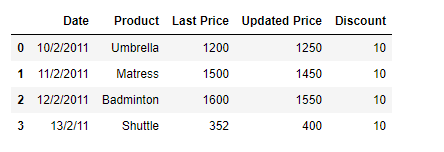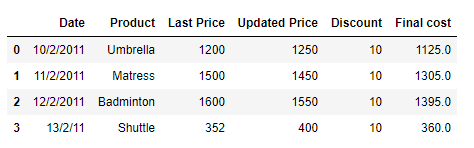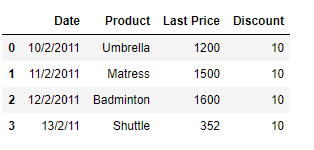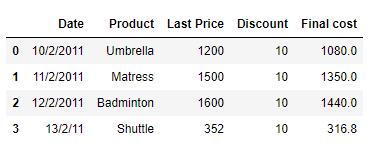GeeksforGeeks App
Open AppBrowser
Continue

# Conditional operation on Pandas DataFrame columns

Suppose you have an online store. The price of the products is updated frequently. While calculating the final price on the product, you check if the updated price is available or not. If not available then you use the last price available. Solution #1: We can use conditional expression to check if the column is present or not. If it is not present then we calculate the price using the alternative column.

## Python3

 `# importing pandas as pd``import` `pandas as pd` `# Create the dataframe``df ``=` `pd.DataFrame({``'Date'``:[``'10/2/2011'``, ``'11/2/2011'``, ``'12/2/2011'``, ``'13/2/2011'``],``                   ``'Product'``:[``'Umbrella'``, ``'Mattress'``, ``'Badminton'``, ``'Shuttle'``],``                   ``'Last Price'``:[``1200``, ``1500``, ``1600``, ``352``],``                   ``'Updated Price'``:[``1250``, ``1450``, ``1550``, ``400``],``                   ``'Discount'``:[``10``, ``10``, ``10``, ``10``]})` `# Print the dataframe``print``(df)`

Output :Now we will check if the updated price is available or not. If not available then we will apply the discount of 10% on the ‘Last Price’ column to calculate the final price.

## Python3

 `# Check if the updated price is available or not``if` `'Updated Price'` `in` `df.columns:``    ``df[``'Final cost'``] ``=` `df[``'Updated Price'``] ``-` `(df[``'Updated Price'``]``*``0.1``)` `else` `:``    ``df[``'Final cost'``] ``=` `df[``'Last Price'``] ``-` `(df[``'Last Price'``]``*``0.1``)` `# Print the Dataframe``print``(df)`

Output :As we can see in the output, as the ‘Update Price’ column was available, so the ‘Final Cost’ has been calculated on the updated price.   Now let’s consider a scenario when the ‘Updated Price’ is not available.

## Python3

 `# importing pandas as pd``import` `pandas as pd` `# Create the dataframe``df ``=` `pd.DataFrame({``'Date'``:[``'10/2/2011'``, ``'11/2/2011'``, ``'12/2/2011'``, ``'13/2/2011'``],``                   ``'Product'``:[``'Umbrella'``, ``'Mattress'``, ``'Badminton'``, ``'Shuttle'``],``                   ``'Last Price'``:[``1200``, ``1500``, ``1600``, ``352``],``                   ``'Discount'``:[``10``, ``10``, ``10``, ``10``]})` `# Print the dataframe``print``(df)`

Output :Now we will check if the updated price is available or not. If not available then we will apply the discount of 10% on the ‘Last Price’ column to calculate the final price.

## Python3

 `# Check if the updated price is available or not``if` `'Updated Price'` `in` `df.columns:``    ``df[``'Final cost'``] ``=` `df[``'Updated Price'``] ``-` `(df[``'Updated Price'``]``*``0.1``)` `else` `:``    ``df[``'Final cost'``] ``=` `df[``'Last Price'``] ``-` `(df[``'Last Price'``]``*``0.1``)` `# Print the Dataframe``print``(df)`

Output :Solution #2: We can use Python’s issubset() function to check if the desired columns are present in the set or not.

## Python3

 `# importing pandas as pd``import` `pandas as pd` `# Create the dataframe``df ``=` `pd.DataFrame({``'Date'``:[``'10/2/2011'``, ``'11/2/2011'``, ``'12/2/2011'``, ``'13/2/2011'``],``                   ``'Product'``:[``'Umbrella'``, ``'Mattress'``, ``'Badminton'``, ``'Shuttle'``],``                   ``'Last Price'``:[``1200``, ``1500``, ``1600``, ``352``],``                   ``'Updated Price'``:[``1250``, ``1450``, ``1550``, ``400``],``                   ``'Discount'``:[``10``, ``10``, ``10``, ``10``]})` `# Print the dataframe``print``(df)`

Output :Now we will check if the updated price is available or not. If not available then we will apply the discount of 10% on the ‘Last Price’ column to calculate the final price.

## Python3

 `# Check if the updated price is available or not``if` `{``'Updated Price'``, ``'Discount'``}.issubset(df.columns):``    ``df[``'Final cost'``] ``=` `df[``'Updated Price'``] ``-` `(df[``'Updated Price'``]``*``0.1``)` `elif` `{``'Last Price'``, ``'Discount'``}.issubset(df.columns):``    ``df[``'Final cost'``] ``=` `df[``'Last Price'``] ``-` `(df[``'Last Price'``]``*``0.1``)` `# Print the Dataframe``print``(df)`

Output :As we can see in the output, as the ‘Update Price’ column was available, so the ‘Final Cost’ has been calculated on the updated price. Now let’s consider a scenario when the ‘Updated Price’ is not available.

## Python3

 `# importing pandas as pd``import` `pandas as pd` `# Create the dataframe``df ``=` `pd.DataFrame({``'Date'``:[``'10/2/2011'``, ``'11/2/2011'``, ``'12/2/2011'``, ``'13/2/2011'``],``                   ``'Product'``:[``'Umbrella'``, ``'Mattress'``, ``'Badminton'``, ``'Shuttle'``],``                   ``'Last Price'``:[``1200``, ``1500``, ``1600``, ``352``],``                   ``'Discount'``:[``10``, ``10``, ``10``, ``10``]})` `# Print the dataframe``print``(df)`

Output :Now we will check if the updated price is available or not. If not available then we will apply the discount of 10% on the ‘Last Price’ column to calculate the final price.

## Python3

 `# Check if the updated price is available or not``if` `{``'Updated Price'``, ``'Discount'``}.issubset(df.columns):``    ``df[``'Final cost'``] ``=` `df[``'Updated Price'``] ``-` `(df[``'Updated Price'``]``*``0.1``)` `elif` `{``'Last Price'``, ``'Discount'``}.issubset(df.columns):``    ``df[``'Final cost'``] ``=` `df[``'Last Price'``] ``-` `(df[``'Last Price'``]``*``0.1``)` `# Print the Dataframe``print``(df)`

Output :As we can see in the output, as the ‘Update Price’ column was not available, so the ‘Final Cost’ has been calculated on the basis of last price.

My Personal Notes arrow_drop_up